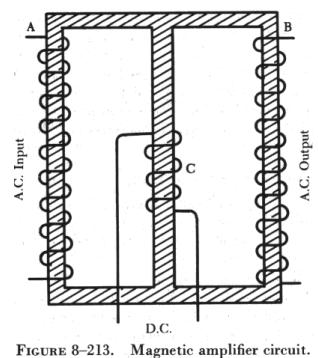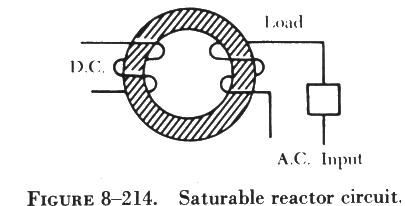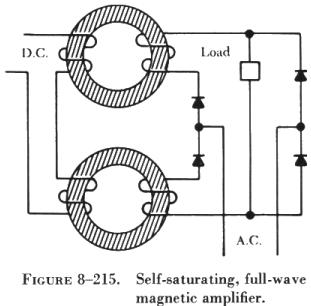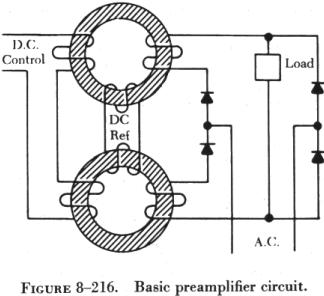MAGNETICAMPLIFIERS MAGNETIC AMPLIFIERS

 The magnetic amplifier is a control device being employed at an increasing rate in many aircraft electrical and electronic systems. This is because of its ruggedness, stability, and safety in comparison to vacuum tubes. The principles on which the magnetic amplifier operates can best be explained by reviewing the operation of a simple transformer. If an ac voltage is applied to the primary of an iron core transformer, the iron core will be magnetized and demagnetized at the same frequency as that of the applied voltage. This, in turn, will induce a voltage in the transformer secondary. The output voltage across the terminals of the secondary will depend on the relationship of the number of turns in the primary and the secondary of the transformer. The iron core of the transformer has a saturation point after which the application of a greater magnetic force will produce no change in the intensity of magnetization. Hence, there will be no change in transformer output, even if the input is greatly increased. The magnetic amplifier circuit in figure 8-213 will be used to explain how a simple magnetic amplifier functions.Assume that there is 1 ampere of current in coil A, which has 10 turns of wire. If coil B has 10 turns of wire, an output of 1 ampere will be obtained if coil B is properly loaded. By applying direct current to coil C, the core of the magnetic amplifier coil can be further magnetized. Assume that coil C has the proper number of turns and, upon the application of 30 milliamperes, that the core is magnetized to the point where 1 ampere on coil A results in only 0.24 ampere output from coil B.

By making the dc input to coil C continuously variable from 0 to 30 milliamperes and maintaining an input of 1 ampere on coil A, it is possible to control the output of coil B to any point between 0.24 ampere and 1 ampere in this example. The term "amplifier" is used for this arrangement because, by use of a few milliamperes, control of an output of 1 or more amperes is obtained.The same procedure can be used with the circuit shown in figure 8-214.

By controlling the extent of magnetization of the iron ring, it is possible to control the amount of current flowing to the load, since the amount of magnetization controls the impedance of the ac input winding. This type of magnetic amplifier is called a simple saturable reactor circuit.

Adding a rectifier to such a circuit would remove half the cycle of the ac input and permit a direct current to flow to the load. The amount of dc flowing in the load circuit is controlled by a dc control winding (sometimes referred to as bias). This type of magnetic amplifier is referred to as being self-saturating.

In order to use the full ac input power, a circuit such as that shown in figure 8-215 may be used. This circuit uses a fullwave bridge rectifier. The load will receive a controlled direct current by using the full ac input. This type of circuit is known as a selfsaturating, fullwave magnetic amplifier.In figure 8-216 it is assumed that the dc control winding is supplied by a variable source, such as a sensing circuit. In order to control such a source and use its variations to control the ac output, it is necessary to include another dc winding that has a constant value. This winding, referred to as the reference winding, magnetizes the magnetic core in one direction.The dc control winding, acting in opposition to the reference winding, either increases (degenerative) or decreases (regenerative) the magnetization of the core to change the amount of current flowing through the load. This is essentially a basic preamplifier.